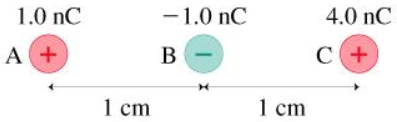# Problem: A. What is the magnitude of the electric force on charge A in the figure?F=______NB. What is the direction of the electric force on charge A in the figure? Choose the best answer.(a) to the left(b) to the right(c) the force is zero

###### FREE Expert Solution

A. Electric force at point A is due to the charges at point B and C.

We apply Coulomb's law between points A and B and again between points A and C.

$\overline{){\mathbf{F}}{\mathbf{=}}\frac{{\mathbf{kq}}_{\mathbf{1}}{\mathbf{q}}_{\mathbf{2}}}{{\mathbf{r}}^{\mathbf{2}}}}$

Between A and B

$\begin{array}{rcl}{\mathbf{F}}_{\mathbf{A}\mathbf{B}}& \mathbf{=}& \frac{{\mathbf{kq}}_{\mathbf{A}}{\mathbf{q}}_{\mathbf{B}}}{{{\mathbf{r}}_{\mathbf{AB}}}^{\mathbf{2}}}\end{array}$

• k = 8.99 × 109Nm2/C2 (Coulomb's constant)
• qA = 1.0 × 10-9 C
• qB = - 1.0 × 10-9 C
• rAB = 1 × 10-3 m

Substituting:

$\begin{array}{rcl}{\mathbf{F}}_{\mathbf{AB}}& \mathbf{=}& \frac{\mathbf{\left(}\mathbf{8}\mathbf{.}\mathbf{99}\mathbf{×}{\mathbf{10}}^{\mathbf{9}}{\mathbf{Nm}}^{\mathbf{2}}\mathbf{/}{\mathbf{C}}^{\mathbf{2}}\mathbf{\right)}\mathbf{\left(}\mathbf{1}\mathbf{.}\mathbf{0}\mathbf{×}{\mathbf{10}}^{\mathbf{-}\mathbf{9}}\mathbf{C}\mathbf{\right)}\mathbf{\left(}\mathbf{-}\mathbf{1}\mathbf{.}\mathbf{0}\mathbf{×}{\mathbf{10}}^{\mathbf{-}\mathbf{9}}\mathbf{C}\mathbf{\right)}}{{\mathbf{\left(}\mathbf{1}\mathbf{.}\mathbf{0}\mathbf{×}{\mathbf{10}}^{\mathbf{-}\mathbf{3}}\mathbf{m}\mathbf{\right)}}^{\mathbf{2}}}\\ & \mathbf{=}& \frac{\mathbf{\left(}\mathbf{8}\mathbf{.}\mathbf{99}\mathbf{×}{\mathbf{10}}^{\mathbf{9}}\mathbf{N}\overline{){\mathbf{m}}^{\mathbf{2}}}\mathbf{/}\overline{){{\mathbf{C}}}^{{\mathbf{2}}}}\mathbf{\right)}\mathbf{\left[}\mathbf{\left(}\mathbf{1}\mathbf{.}\mathbf{0}\mathbf{×}{\mathbf{10}}^{\mathbf{-}\mathbf{9}}\mathbf{\right)}\mathbf{\left(}\mathbf{-}\mathbf{1}\mathbf{.}\mathbf{0}\mathbf{×}{\mathbf{10}}^{\mathbf{-}\mathbf{9}}\mathbf{\right)}\mathbf{\right]}\overline{){\mathbf{C}}^{\mathbf{2}}}}{\mathbf{1}\mathbf{.}\mathbf{0}\mathbf{×}{\mathbf{10}}^{\mathbf{-}\mathbf{6}}\overline{){\mathbf{m}}^{\mathbf{2}}}}\end{array}$

FAB =  - 8.99 × 10-3 N

95% (381 ratings)###### Problem DetailsA. What is the magnitude of the electric force on charge A in the figure?

F=______N

B. What is the direction of the electric force on charge A in the figure? Choose the best answer.

(a) to the left

(b) to the right

(c) the force is zero